## Why Fractal Shape Antennas ?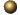Antennas are essentially narrowband devices. Their behavior is highly dependent on the antenna size to the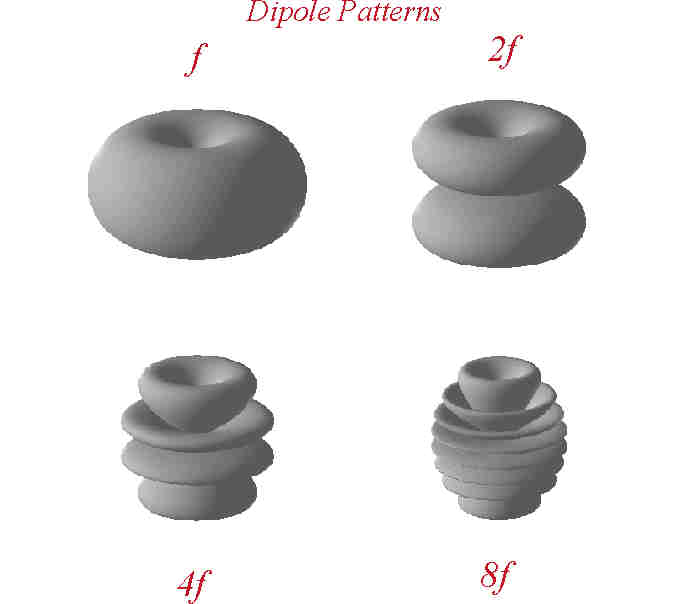operating wavelength ratio. This means that for a fixed antenna size, the main antenna parameters (gain, input impedance, pattern shape and secondary lobe level and distribution) will suffer strong variations when changing the operating frequency. For instance, the picture on the right shows the evolution with frequency of the radiation pattern of a classical common antenna (a linear dipole). Each time frequency is doubled, several grating lobes appear, modifying the way the antenna spreads power into space.

Analogously, the frequency dependence also implies that an antenna has to keep a minimum size relative to wavelength to operate efficiently. That is, given a particular frequency, the antenna can not be made arbitrarily small: it usually has to keep a minimum size, typically on the order of a quarter wavelength. These well known results have been constraining for decades the antenna performance in telecommunication systems, and they have been the object of an intensive research with some successful results. However, the size to wavelength dependence is still a problem in many systems where former antenna designs are not particularly suitable. In that sense, the fractal design of antennas and arrays can help in dealing with the problem by contributing with a huge, rich variety of geometrical shapes with some stonishing properties. In this website the reader will find some examples of succesful designs of fractal multiband and small antennas.Fractals are a whole new set of geometrical objects featuring two main common properties: self-similarity and fractional dimension. The first property is rather intuitive and readily comes out from looking to a fractal object. Roughly speaking, an object is said to be self-similar when it is enterely constructed by many copies of itself at different scales (see the example on the left side of the page). On the other hand, the fractional dimensional property is less intuitive but it can be explained by looking to the example of the figure.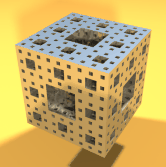The construction of such a fractal shape (named the Menger Sponge) starts from drilling a square hole on the center of each side of a solid cube. The next step consists on drilling 8 new holes around the main one, each of them being 3 times smaller than the original one. If that procedure (burning 8 new holes around each one from the former stage) were carried out an infinite number of times, the ideal self-similar fractal shape would be obtained. The ideal object would contain an infinite number of copies of itself with a characteristic scale factor of 3 relating the sizes of them all. Somehow, the intuition would tell us that such an object is a 3D body, however the volume of the final shape after the infinite drilling process vanishes. Nevertheless, the object seems to fill a 3D space better than a simple 2D surface. Actually, it can be mathematically proved that such an object has a dimension of D ~ 2.72, which means that is half-way between a 3D and a 2D object. In other words, the object is a surface that fills a 3D space better than any classical Euclidean surface.The reason why the fractal design of antennas appears as an attractive way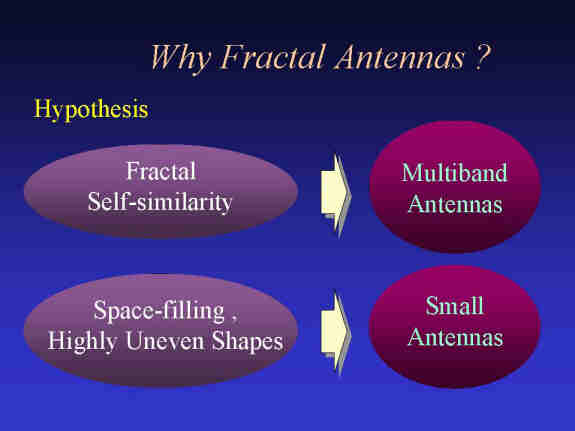to make antennas is two-fold. First because one should expect a self-similar antenna (which contains many copies of itself at several scales) to operate in a similar way at several wavelengths. That is, the antenna should keep similar radiation parameters through several bands. Second, because the space-filling properties of some fractal shapes (the fractal dimension) might allow fractally shaped small antennas to better take advantage of the small surrounding space. Such basic concepts have been already confirmed by the examples you will find here in the Fractal AntennasTM Gallery.(c) by Carles Puente Baliarda, April 1998. For any comments please contact at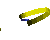webmaster